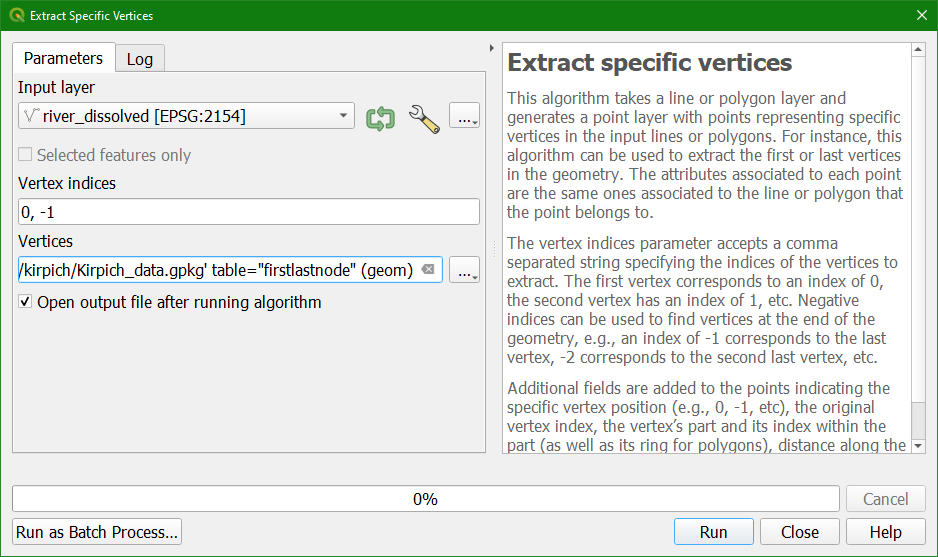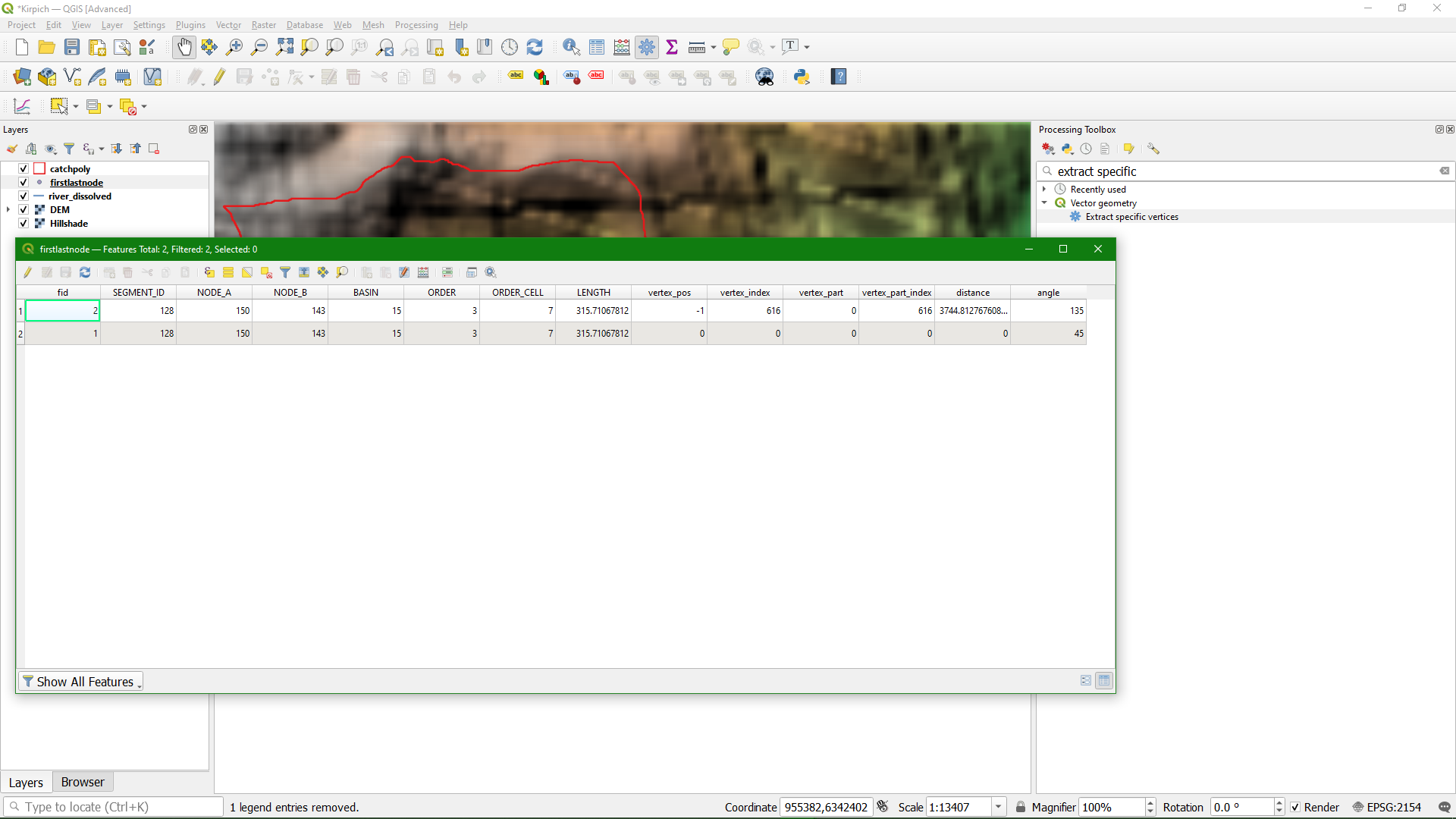Tutorial: Calculate time of concentration (Kirpich)

4. Find the first and last node of the river

In this step we're going to find the first and last node of the river. For the Kirpich equation we later need the distance between these points and their elevation difference.

1. Open the Processing Toolbox: from the main menu choose Processing | Toolbox.

2. click on the tool Vector Geometry | Extract specific verticesThis tool can be used to extract specific vertices.The first vertex corresponds to an index of 0, the second vertex has an index of 1, etc. Negative indices can be used to find vertices at the end of the geometry, e.g., an index of -1 corresponds to the last vertex, -2 corresponds to the second last vertex, etc.

3. In the Extract Specific Vertices dialogue choose river_dissolved as Input layer, for Vertex indices type 0, -1 and save it to the Kirpich_data GeoPackage with the name firstlastnode. Click Run.4. Click Close after processing.

5. Click right on the firstlastnode layer and choose Open Attribute Table.You can see in the field vertex_pos the index of the vertex and in the distance field the distance from the first node in meters. So here the distance of the last node is 3745 m. We need that value in the equation.
We also need the elevation difference between the first and last node. In the next step we're going to sample the elevation on the two nodes.